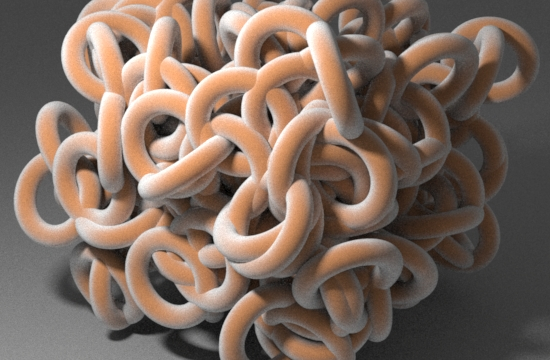### OSLEdge Effects

#### Introduction

This tutorial shows how to apply interesting shading effects to different parts of a curved surface. The technique is demonstrated by a shader called `Velvet`. The effect of the shader is similar to soft rim lighting but without the use of a light source. The shader outputs a color that is a mixture of two inputs colors. At each shading point the mixing of the colors is controlled by the magnitude of the angle between the viewing vector and the surface normal and a parameter called rim_width.Rollover - without and with displacement The shader, listing 1, also outputs a float called resultF which can be used to achieve some interesting displacement effects.

Listing 1 - Velvet.osl

 ```shader Velvet( float rim_width = 0.2 [[ string label = "Rim Width", ]], color basecolor = color(1,1,1) [[ string label = "Base Color", string widget = "checkBox", ]], color rimcolor = color(1,0,0) [[ string label = "Rim Color", ]], output color resultRGB = 0, output float resultF = 0) { vector i = normalize(I); vector n = normalize(N); float d = fabs(dot(-i, n)); d = smoothstep(rim_width, 1.0, d); resultRGB = mix(rimcolor, basecolor, d); resultF = d - 0.5; }```

#### Using the Viewing Vector and Surface Normal

The following illustration shows how the angle between the surface normal and the line-of-sight of the camera ie. the viewing vector, changes from 0.0 degrees at the center of the object (location A) to 90.0 degrees at the rim (location B).The angle is calculated using vector multiplication known as the dot product (also called the scalar or inner product). The OSL function `dot()` returns a value between 1.0 when the shading point is directly facing the camera, -1.0 when the shading point is directly facing away from the camera and 0.0 when the shading point at the the silhouette edge of a curved object.

In other words, the `dot()` function returns an angle measured not in degrees but as the cosine of the angle. The `smoothstep()` function is used to remap the values of the dot product so the artist can control the "width" of the edge effect.